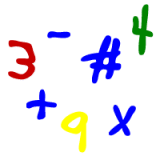Parents and Teachers: Support Ducksters by following us onor.

Kids Math

ExponentsSkills needed:
Multiplication

Using exponents is just a short way of saying that you want to multiply something by itself a number of times. Let's say for example that you want to do the following:

4 x 4 x 4

This could be written with exponents and would look like this:

43

They both equal the same thing which is 64, but the exponent way is shorter and easier to write. This comes in real handy when you want to multiply something a lot of times.

Terminology

In the example above, 43, 4 is called the "base" and "3" is called the "exponent". It is often described as "4 to the power of 3". So the exponent is also sometimes called "the power of" number.

Before we move on, let's do one more simple exponent example:

24 = 16

We got this by multiplying 2 x 2 x 2 x 2.

2x2=4
4x2=8
8x2=16

Special Exponents

There are some special exponents that we can study next:

Squared

When something has an exponent of 2, we call it squared. The name comes from finding the area of a square.

Cubed

When something has an exponent of 3, we called it cubed. This name comes from finding the area of a cube.

Tricky stuff

The first tricky thing to watch out for is an exponent of 0. ANY time there is an exponent of 0, the answer is 1. For example:

40 = 1

Even a long crazy looking equation like (4y-7+x+2z)0 still equals 1.

Harder stuff

Let's say we have:

43 x 42

It turns out this is the same as 43+2 or 45

In the case where the bases are the same we can add the exponents during multiplication.

(43) 2

This is the same as 42x3 or 46. When we have an exponent on top of an exponent, then we multiply the exponents.

More Algebra Subjects
Algebra glossary
Exponents
Linear Equations - Introduction
Linear Equations - Slope Forms
Order of Operations
Ratios
Ratios, Fractions, and Percentages
Solving Algebra Equations with Addition and Subtraction
Solving Algebra Equations with Multiplication and Division

Back to Kids Math

Back to Kids StudyFollow us onor